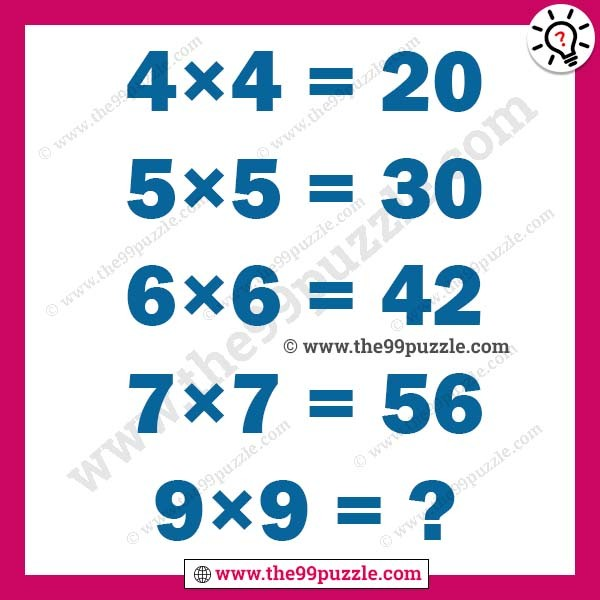# Brain test logical math puzzle with answer – Puzz192

Can you solve the brain test logical math puzzle? If you are a genius in math puzzles you can easily solve this puzzle. In this brain puzzle simply apply logic and solve the math puzzle. Can you calculate the math puzzle answer?

4×4=20

5×5=30

6×6=42

7×7=56

9×9=?###### Explanation:

4×4=4×4+4=20

5×5=5×5+5=30

6×6=6×6+6=42

7×7=7×7+7=56

9×9=9×9+9=90

1.